# Tensor Calculus

Also found in: Dictionary, Thesaurus, Medical.
Related to Tensor Calculus: Vector calculus

## tensor calculus

[′ten·sər ‚kal·kyə·ləs]
(mathematics)
McGraw-Hill Dictionary of Scientific & Technical Terms, 6E, Copyright © 2003 by The McGraw-Hill Companies, Inc.
The following article is from The Great Soviet Encyclopedia (1979). It might be outdated or ideologically biased.

## Tensor Calculus

a mathematical theory that studies the properties of, and operations on, mathematical objects called tensors. Tensor calculus is an extension and generalization of vector calculus and matrix theory. It has many uses in such disciplines as differential geometry, the theory of Riemannian manifolds, relativity, mechanics, and electrodynamics.

Coordinate systems are a common means of describing many physical and geometric facts. In this approach objects are labeled by single numbers or by sets of numbers, and relations among objects are described by equations connecting the respective numbers or sets of numbers. Scalars, for example, mass and temperature, are described by single numbers that do not change upon transition from one coordinate system to another. (We are treating physical phenomena here from the standpoint of classical physics.) Vectors, for example, force and velocity, are given by three numbers, which are called the components of the vector; these three numbers change according to a definite law upon transition from one coordinate system to another. Scalars and vectors are the simplest members of a class of objects called tensors that appear in many physical and geometric problems. The more complex tensors are described in each coordinate system by a set of numbers—the components of the tensor—that change upon transition from one coordinate system to another according to a law more complex than the corresponding law for vectors. When we use a coordinate system, then, in addition to the numbers that describe an object or physical phenomenon, there appear numbers describing the relation of the object or phenomenon to that system.

For example, consider the numbers Jij, i, j = 1, 2, 3, where Jii is the axial moment of inertia of a rigid body with respect to the xi,-axis, and Jii. i ≠ j, are the negatives of the products of inertia. A change of coordinates induces a change in the position of the xi-axis and thus a change in the value of the axial moment of inertia Jii. It follows that Jii is not a physical magnitude having significance independent of the coordinate system. One manifestation of this fact is that knowledge of the value of Jii in one coordinate system is not sufficient to determine it in another. On the other hand, the totality of numbers Jii has significance that is independent of the choice of a coordinate system: if we know the Jii in one rectangular coordinate system, then we can find their values in any other rectangular coordinate system by means of the formula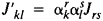. where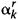and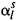are certain numbers. The omission of summation signs is a standard convention. If an index appears twice, once as upper and once as lower index, then we sum over that index and assign to it the relevant integral values—in our example the values 1, 2, 3.

Like vector calculus, tensor calculus is a mathematical apparatus that eliminates the effect of the choice of a particular coordinate system in the sense that the components of a tensor in one coordinate system determine its components in any other coordinate system. In the tensor calculus we develop methods for obtaining invariant relations and invariants—that is, relations between tensors, and functions of the tensor components, that are not affected by coordinate changes.

It should be clear by now that one of the fundamental problems of the tensor calculus is the formulation of the laws of mechanics, geometry, and physics in a manner independent of the choice of a coordinate system.

Cartesian tensors. A Cartesian tensor is determined in every rectangular coordinate system in three-dimensional space by 3k numbers

pi1 ... ik ir= 1, 2, 3

which change to the numbers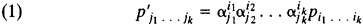when the coordinate system (x1, x2, x3) is changed to the coordinate system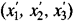. Here,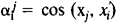. The number k is called the rank of the tensor, and the

pi1 ... ik

are called its components, or coordinates. There are similar definitions of tensors in n-dimensional Euclidean space.

Let us consider some examples of tensors. The components ai, i = 1, 2, 3, of a vector a form a tensor of rank one. The vectors a = {ai} and b = {bi} determine a tensor with components pij = ai bi known as a dyad. If a(x1, x2, x3) is a vector field, then each of its points determines a tensor with components pij = ∂ai/∂Xj known as the derivative da/dr of the vector r = {aj} with respect to the vector r{x1,x2, x3. The numbers Jij mentioned earlier form a tensor of rank two—the inertia tensor.

Tensors of rank two. In addition to tensors of rank one (vectors), the tensors most frequently encountered in applications to mechanics are tensors of rank two.

A tensor is called symmetric if pij = pij and skew symmetric if pij = pji. A symmetric tensor has six essential components. A skew symmetric tensor has three essential components: ω1, = p32, = –p23, ω2= pl3 = –p31 and ω3 = p21 = –p12 (p11 = p22 = p33 = 0). The components ω1, ω2, ω2 and ω3 transform like the components of a pseudovector. In general, pseudovectors, such as angular velocity and the cross product of two vectors, may be regarded as skew symmetric tensors of rank two. The tensor whose components in every coordinate system are p11 = p22 = p33 = 1 and pij = 0 for i ≠ j is called the unit tensor. Its components are denoted by the Kronecker symbol δij. The inertia, stress, and unit tensors are symmetric. Every tensor can be uniquely represented as a sum of a symmetric and a skew symmetric tensor. If a(r) is the displacement vector associated with a small deformation, then the symmetric part of da/dr is called the deformation tensor. Its skew symmetric part is (½) curl a. The tensor da/dr is symmetric only if the field a (r) is irrotational. To the decomposition of the tensor da/dr into a symmetric and a skew symmetric part there corresponds the decomposition of the relative displacement da into a pure deformation and a rotation of the body as a whole.

We recall that invariants of a tensor are functions of its components that do not depend on the choice of a coordinate system. An example of an invariant is the trace p11 + p22 + p33 of a tensor. For the inertia tensor, the trace is twice the polar moment of inertia with respect to the origin; for the tensor da/dr. the trace is the divergence of the vector field a(r).

Affine tensors. In many problems it is necessary to consider tensors in affine coordinate systems. In such coordinate systems, the axes need not be orthogonal, and the units of length on different axes need not be equal. The connection between two affine coordinate systems is determined by the components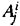of the new basis vectors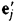relative to the old basis vectors ei and by the components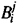of the basis vectors ei, relative to the basis vectors. Accordingly, we may distinguish covariant, contravariant, and mixed tensors: the transformation laws of covariant tensors involve the numbers. the transformation laws of contravariant tensors involve the numbers. and the transformation laws of mixed tensors involve theand the. More precisely, a mixed tensor of rank r + s that is s times covariant and r times contravariant [briefly, a tensor of type (s, r)] is specified in each coordinate system by 3r+s numbers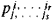that transform as a result of a change of coordinates according to the rule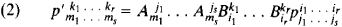In the case of the rectangular coordinate system,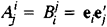. For Cartesian tensors, consequently, we need not distinguish between covariant (lower) and contravariant (upper) indices.

The coefficients of the equation of a quadric surface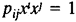form a covariant tensor of rank two, and the elements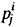of the matrix of a linear transformation form a once covariant and once contravariant tensor. A system of three numbers x1, x2, x3 that transform in the manner of the components of a vector x = xiei forms a once contravariant tensor, and a system of numbers that transform in the manner of a scalar product xi = xei forms a once covariant tensor. Relative to affine coordinate transformations, the Kronecker symbol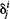is a mixed tensor—hence the upper and lower indices, in contrast to the situation discussed above in the section “Tensors of rank two.” The numbers gij = eiej, where the ei form a basis, are the covariant metric tensor. The length of a vector x = xiei is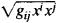and the scalar product of two vectors x and y is gijxiyj. The numbers gij with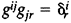form the contravariant metric tensor.

There are analogous definitions of tensors in n-dimensional space. The components of p-vectors are important examples of tensors in n-dimensional space.

The order of the indices is an essential part of the definition of a tensor. In general, a permutation of the indices of a tensor results in a change of its components. A tensor is said to be symmetric with respect to a set of indices on the same level if the interchange of any two of these indices leaves unaltered all of its components. It is skew symmetric with respect to these indices if such an interchange changes the algebraic sign of its components. More generally, any invariant linear dependence between the components of a tensor is called a symmetry condition of the tensor.

Operations on tensors. The four basic operations on tensors are addition, multiplication, contraction with respect to two or more indices, and transposition of indices. Since a tensor is given by its components in different coordinate systems, operations on tensors are defined by formulas that give in each coordinate system the components of the result of the operation in terms of the components of the tensors involved in the operation. Of course, the result must be a tensor.

ADDITION OF TENSORS. The sum of two tensors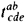and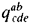of the same type—that is, with the same number of upper indices and the same number of lower indices, is the tensor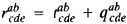.

MULTIPLICATION OF TENSORSS. The product of two tensors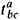and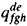(possibly of different types) is the tensor with components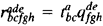. In general, the product of a tensor of rank zero, that is, a scalar λ, by a tensor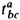is the tensor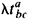.

CONTRACTION OF A TENSOR. The result of the contraction of a tensor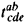with respect to the upper index a and the lower index d is the tensor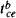with components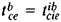. For example, contraction of a matrix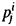with respect to the indices i and j yields the trace of the matrix, and the biscalar product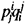of the tensorsand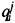is the result of contraction of their product with respect to all indices. Contraction of a tensor with respect to all indices yields an invariant.

TRANSPOSITION OF INDICES. If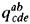and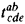are tensors such that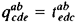then we say thatobtained fromby transposing the indices c and e. The transposed indices must be on the same level.

Tensor analysis. In applications we usually encounter tensor fields rather than tensors. In the study of elastic deformations, for example, the deformation and stress tensors at all points of a body are considered. Given a rectangular coordinate system in space, we can regard a tensor field T(P) as the totality of functions ti1 ... ik(xl, x2, x3), defined at the points P(x1, x2, x3) of some region, that transform as a result of a change of coordinates according to formulas of type (1). Then each partial derivative δti1 ... ik/δXi forms a tensor of rank one higher than the original tensor. For example, differentiation of a scalar field yields the gradient field, and differentiation of the gradient field yields the field of the symmetric tensor 2f/∂xi∂xi.

Tensor analysis makes use not only of Cartesian and affine coordinates but also of arbitrary, sufficiently smooth curvilinear coordinates xi. In the neighborhood of each point such coordinates can be replaced by affine coordinates using as basis vectors the partial derivatives ∂r/∂xi of the radius vector r at P. The scalar products eiej are then equal to the components of the metric tensor gij at P, and the length of an infinitesimal vector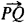, P(xi), Q(xi + dxi). is given by the equation ds2 = gijdxidxj. The metric forms for curvilinear and affine coordinates, consequently, are equal up to order two. Thus, at any point there is a local affine coordinate system, and the components of a tensor field relate to these systems. If the curvilinear coordinates (x1, ..., xn) change to (y1, . . ., yn). then the local coordinate systems change also, and the local basis vectors transform in accordance with the formula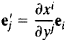In other words, the coefficients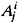and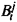vary from point to point, and their respective values are ∂xi/∂yi and ∂yi/∂xi. For this reason, we define a tensor field relative to a curvilinear coordinate system as a system of functions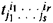of the curvilinear coordinates. These functions are defined in some region and transform as a result of a transition from one curvilinear coordinate system to another according to equation (2), where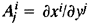and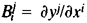. In this case, the partial derivatives of the field components with respect to the coordinates xi do not form a tensor field. The reason for this fact is that upon transition from point to point there is a change not only in the tensor components but also in the local coordinate system to which the tensor relates.

It follows that in order to determine the increment of a tensor we must consider not only the increments of its components due to the transition from the point P(xi) to the infinitesimally close point P(xi + dxi) but also the change in the local coordinate system. In other words, we must not identify the components of the increment of a tensor with the increments of its components. For example, in the case of vector fields u(P), where u has contravariant components ui, the increment of the vector field is given, apart from higher-order terms, by the equation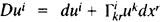. Here,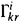is the Christoffel symbol, which is linked to the metric tensor gij by means of the relation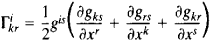It should be noted that the Christoffel symbols are not tensors. The summand dui takes into account the dependence of the components of the increment of the tensor on the increments of its components, and the summand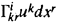takes into account the dependence of the components of the increment of the tensor on the change of coordinate system associated with the transition from point to point.

The vector Dui is called the covariant, or absolute, differential of the vector field u(P), and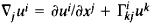is the covariant, or absolute, derivative of the field. Similarly, the covariant derivative of a covariant vector field is equal to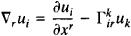The covariant derivative of a tensor field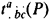is defined by the equation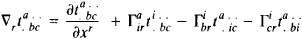The covariant derivative of a tensor field is a tensor field whose covariant rank is one greater than the covariant rank of the original field. If the curvilinear coordinates are rectangular, then co-variant differentiation reduces to ordinary differentiation—that is, to the operation of forming the field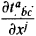In this case, the Christoffel symbols vanish.

For sums and products of tensors, the rules of covariant differentiation coincide with those of ordinary differentiation. Covariant differentiation commutes with contraction. Also, covariant differentiation is commutative in the sense that Δr, ΔmT = ΔmΔrT. It should be noted that the covariant derivative of the metric tensor is zero.

Historical survey. The development of the theory of algebraic forms and the theory of quadratic differential forms in the 19th century prepared the ground for the tensor calculus. The work in the theory of quadratic differential forms was directly related to differential geometry, in particular, the geometry of surfaces (K. Gauss) and the geometry of n-dimensional metric spaces (G. Riemann).

The modern form of the tensor calculus is due to the Italian mathematician C. Ricci and is sometimes called the Ricci calculus. Ricci’s ideas attracted little attention until the appearance of A. Einstein’s general theory of relativity in 1916. The mathematics of the theory of relativity is the mathematics of the tensor calculus.

### REFERENCES

Kochin, N. E. Vektornoe ischislenie i nachala tenzornogo ischisleniia. 9th ed. Moscow, 1965.
Rashevskii, P. K. Rimanova geometriia i tenzornyi analiz, 3rd ed. Moscow, 1967.
Schouten, J. A. Tenzornyi analiz dlia fizikov. Moscow, 1965. (Translated from English.)
McConnell, A. J. Vvedenie v tenzornyi analiz. Moscow, 1963. (Translated from English.)
Sokolnikoff, I. S. Tenzornyi analiz. Moscow, 1971. (Translated from English.)
Based on material from TENSOR CALCULUS in the second edition of the Bol’shaia Sovetskaia Entsiklopediia
The Great Soviet Encyclopedia, 3rd Edition (1970-1979). © 2010 The Gale Group, Inc. All rights reserved.
References in periodicals archive ?
of Warwick) keep in mind fields in which the modeling and simulation of materials with significant coupling and thermal effects as they explain tensor algebra and tensor calculus, then give comprehensive coverage of continuum mass and force concepts, kinematics, balance laws, isothermal fluid and solid mechanics and thermal fluid and solid mechanics.
Appendices give an overview of aspects of Ricci flow, aspects of geometric analysis related to Ricci flow, and tensor calculus on the frame bundle.
He takes an openly didactic approach, and does not hesitate to make those new to the theory as comfortable as possible, covering the mathematical preliminaries first by describing tensors in amorphous spaces and tensors in Riemann spaces, then introduces general relativity in terms of basic theories, Schwarzchild's metric, classical texts and complements of tensor calculus and general relativity.
Coverage includes the basics of various geometries in linear spaces, the geometry of two-dimensional manifolds, basics of topology of smooth manifolds, Lie groups, classical tensor algebra and tensor calculus, differential forms theory, the Riemannian theory of connections and curvature, conformal geometry, complex geometry, Morse theory and Hamiltonian formalism, Poisson and Lagrange manifolds, multidimensional variational problems, and geometric fields in physics.

Site: Follow: Share:
Open / Close